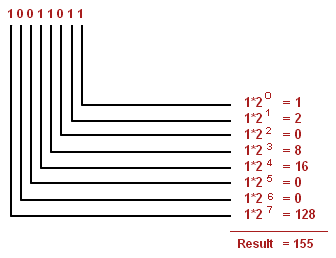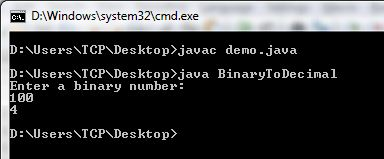# Program to Convert Binary to Decimal in Java

Here you will get program to convert binary to decimal in Java.

There are mainly two ways to convert a binary number to decimal number in Java.

1. By using parseInt() method of Integer class.
2. By using user defined logic.

## Program to Convert Binary to Decimal in Java

By using Integer.parseInt()

Integer.parseInt() method takes two arguments. First argument is a string and second argument is the base or radix in which we have to convert the number. The output is the integer represented by the string argument in the specified radix. Below is the program for it.

Without using Integer.parseInt()

In this method we have to define our own logic for converting binary number to decimal. The approach that we will use here is mentioned in below example.Image Source

The program that implements above approach is mentioned below.

OutputIf you found anything missing or incorrect in above programs then please mention it by commenting below.

### 21 thoughts on “Program to Convert Binary to Decimal in Java”

1.import java.util.Scanner;
public class Binary2Decimal{
public static void main(String[] args) {
Scanner no=new Scanner(System.in);
System.out.println(“Enter a binary number”);
String n=no.nextLine();
System.out.println(Integer.parseInt(n, 2));
}
}
Exception in thread “main” java.lang.NumberFormatException: For input string: “2”
at java.lang.NumberFormatException.forInputString(NumberFormatException.java:65)
at java.lang.Integer.parseInt(Integer.java:580)
at binary2decimal.Binary2Decimal.main(Binary2Decimal.java:8)

1.You have to enter binary number, here you have entered decimal number 2 so exception is coming.

1.Oh thanks I am a beginner so didn’t realise that!

2.Nikhil Kulkarni

y dont you restrict users to give binary number input format only

2.import java.util.Scanner;
public class binary__to__decimal__hardwork__of__1_hours30min__and_30_min__of_deciaml_to_binary_total_of_2_hours
{
public static void main(String args [])
{
Scanner sn=new Scanner(System.in);
System.out.println(“Enter a decimal number”);
int n=sn.nextInt();
int e=0;
int d,y,w;
int f=0;

while(n>0)
{
d=n%10;

if(d==0)
{
d=2;
}
e=e*10+d;
n=n/10;
}

while(e>0)
{
w=e%10;
y=e%2;
if(y==0)
{
w=0;
}
f=f*2+w;

e=e/10;

}
System.out.println(“The decimal value is “+f+ ” work hour include of 3 hours in both ☺♥♥☺ “);

} }

2.public class Bin2dec {

/**
* @param args the command line arguments
*/
public static void main(String[] args) {
Scanner s = new Scanner(System.in);
System.out.println(“Enter the Word”);
String str = s.next();
int number;
double digit = 0 , result = 0;
int check = 1;
for(int i = 0; i < str.length() ; i++){
if(str.charAt(i) =='1' || str.charAt(i) =='0'){
check = 1;
}
else{
check = 0;
}
}
if(check == 0){
System.out.println("Error: Invalid Binary String \"" + str + "\"");
}
else if(check == 1){
number = Integer.parseInt(str);
for(int i = 0; i < str.length() ; i++){
digit = number % 10;
number = number / 10;
result = result + (digit * pow(2,i));
}
System.out.println((int)result);
}

1.It’s correct

2.Dear anything multioliwd by 0 is 0 so dont maje afool of urself writing such a lengty program and confusing the beginners the abov prog is already correct

3.Given code is wrong for input 2100
You just need to use break statement after check=0

1.2100 is not a binary number.

4.import java.io.*;
class BinaryToDecimal
{
public static void main(String [] args) throws IOException
{
System.out.println(“Enter a binary a number:”);
char x[] = a.toCharArray();
int power=0;
long decimal=0 , sum=0;
for(int i =x.length-1 ; i>=0;i–)
{

int z = (int)java.lang.Math.pow(2,power);
sum = sum + ((x[i])*(z));
power++;
sum=0;
}
System.out.println(decimal);
}
}

5.hi sir,

System.out.println(Integer.parseInt(n,2));

1.hi Pardeep,

Integer is a class (wrapper class) and parseInt() is a method .
When we convert a String to int data type , we use this method [parseInt()].For Instance-

String number=”1273″;
int number2= Integer.parseInt(number); // “1273” to 1273

so this method will convert a String (that has digits) to an int . and that 2 after comma is the base of that number.
like Integer.parseInt(“1000”,10) denotes that the number has decimal base ,
Integer.parseInt(“1000”) is the same .

but Integer.parseInt(“1000”,2) means that the number has binary base.
and Integer.parseInt(“1000”,8 ) means that the number has octal base
finally Integer.parseInt(“1000”,16) means that the number has hexadecimal base

in all of these above cases the number will be converted to a number in int type ,i.e. to a decimal number.

6.Hi, the program above is correct but the example image is not correct.

7.I’m a beginner, may I ask about the purpose of n=n/10 ?

1.When we divide any number with 10 the last digit of number is removed from result. For example 123/10 = 12, we have to extract digits from last one be one thats why we used n%10 and n/10.

8.Minor fixes:

1.n/=10 is equivalent to n = n/10

9.How to convert binary to Hexadecimal number using inbuit functions:

10.import java.util.Scanner;

class BinaryToDecimal
{
public static void main(String args[])
{
Scanner s=new Scanner(System.in);

System.out.println(“Enter a binary number:”);
int n=s.nextInt();

int decimal=0,p=0;

while(n!=0)
{
decimal+=((n%10)*Math.pow(2,p));
n=n/10;
p++;
}

System.out.println(decimal);
}
}
C:\Users\my\Desktop\j>javac BinaryToDecimal.java
BinaryToDecimal.java:16: error: cannot find symbol
decimal+=((n%10)*Math.pow(2,p));
^
symbol: method pow(int,int)
location: class Math
1 error

11.Hello Sir,
My query is how could I convert a very very large binary number of length of 10^10 in an efficient way otherwise by our simple method it would take many seconds to convert such number.